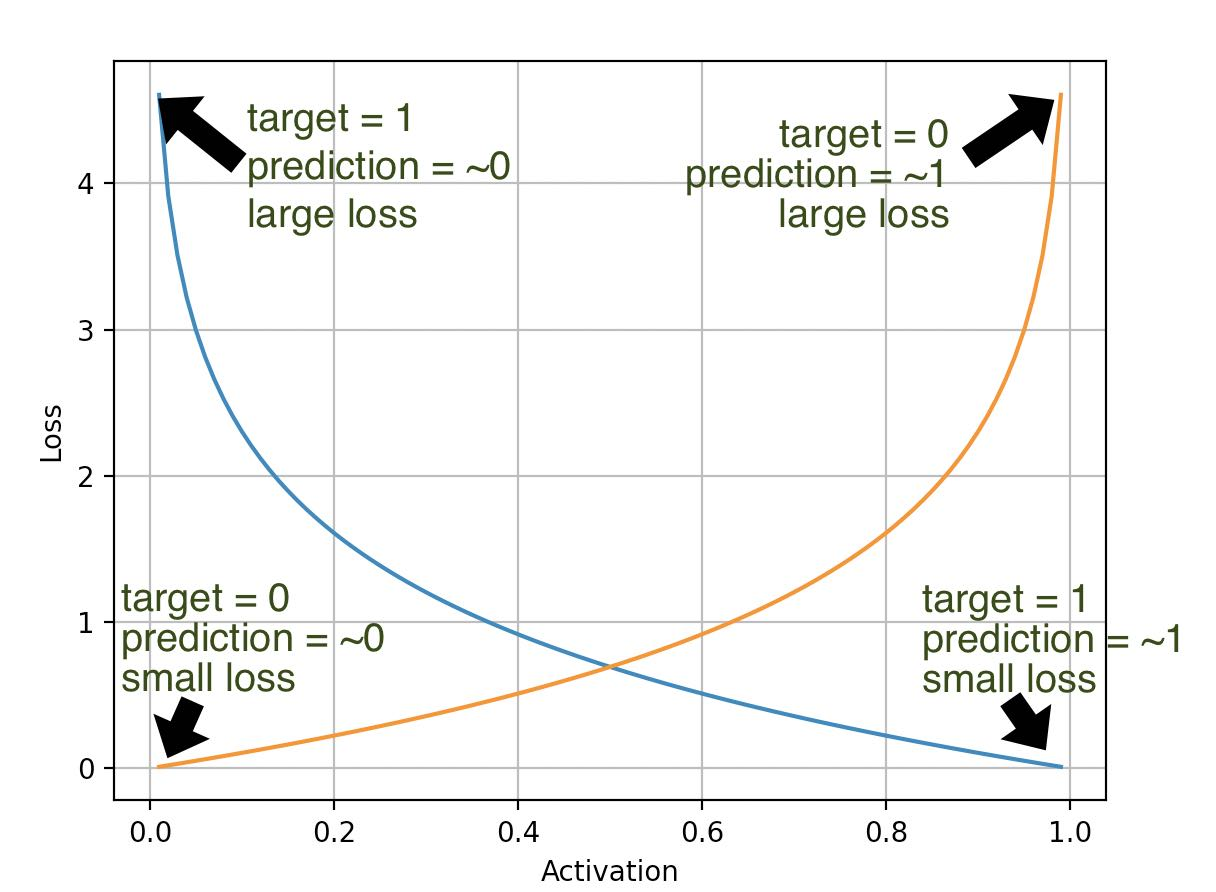If the last layer in your network applies a sigmoid to create a binary classification, how do you measure if the result is any good?

# Logistic regression loss function (log loss).

This code shows how to calculate the loss of a single training sample when the last layer in your neural network apllies a sigmoid function to create a binary classification.

If you are here just for the code, grab this and strip the comments:

```def log_loss(act, target):
""" target == 0 -> -math.log(1 - act)
target == 1 -> -math.log(act) """
return -target * math.log(act) - (1 - target) * math.log(1 - act)```

## Behavior

The goal of the logistic loss function is:

• Create a large loss if the target = 1 and the prediction = ~0
• Create a small loss if the target = 1 and the prediction = ~1
• Create a large loss if the target = 0 and the prediction = ~1
• Create a small loss if the target = 0 and the prediction = ~0

This is what that behavior looks like:If you want a full example that shows the graphic, take this code:

```import math
import matplotlib.pyplot as plt

def log_loss(act, target):
""" target == 0 -> -math.log(1 - act)
target == 1 -> -math.log(act) """
return -target * math.log(act) - (1 - target) * math.log(1 - act)

fig, ax = plt.subplots()

predictions = [r / 100 for r in range(1, 100)]  # -> [.01, 0.02 ... 0.99]

losses_for_target_one = [log_loss(p, 1) for p in predictions]
losses_for_target_zero = [log_loss(p, 0) for p in predictions]

ax.plot(predictions, losses_for_target_one)
ax.plot(predictions, losses_for_target_zero)

ax.set_xlabel("Activation")
ax.set_ylabel("Loss")
plt.grid(True)

plt.show()```
Written by Loek van den Ouweland on 2021-11-30.
Questions regarding this artice? You can send them to the address below.